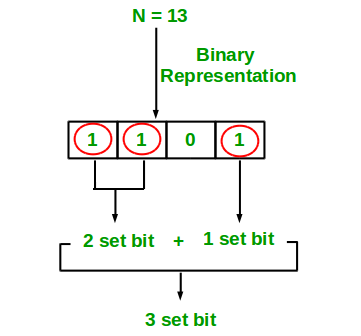# NOTES: EPI Ch4 Primitive Types

## Primitive Types

### 4.1 Parity

Parity fo a binary word is 1 if the number of 1s in the word is odd, o.w. is 0.

def parity(x):  # brute force
result = 0
while x:
result ^= x & 1
x >>= 1
return result  # O(n)

#------------------------ improve--------------------------#
# Trick: x & (x-1) equals x with its lowest set bit erased.#
# e.g. (00101100) & (00101011) = 00101000
#----------------------------------------------------------#

def parity_good(x):
resutl = 0
while x:
result ^= 1
x &= x-1  # drops lowest set bit of x
return result  # O(k) where k is the number of bits set (= number of 1's in the binary number)

## ex
parity(3)

0


### P4_1 count bits

count number of 1s in binary representation of an integer.(img source).

def count_bits(x):
num_bits = 0
while x:
num_bits += x & 1   # go through digit by digit
x >>= 1             # right shift 1 digit
return num_bits         # O(n)

def count_bits_recur(x):

# base case
if (x == 0):
return 0

else:
# if last bit set add 1 else
return (x & 1) + count_bits_recur(x >> 1)  # O(logn)

## ex
count_bits(9)

2

## ex
count_bits_recur(4)

1


Tags:

Updated: